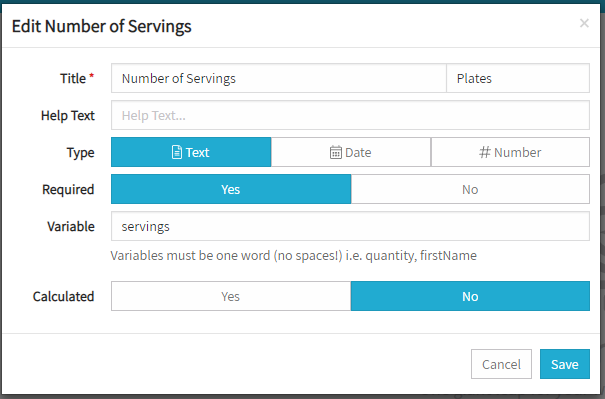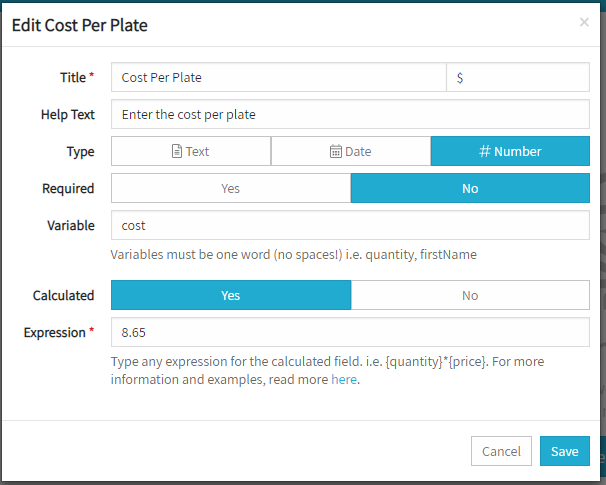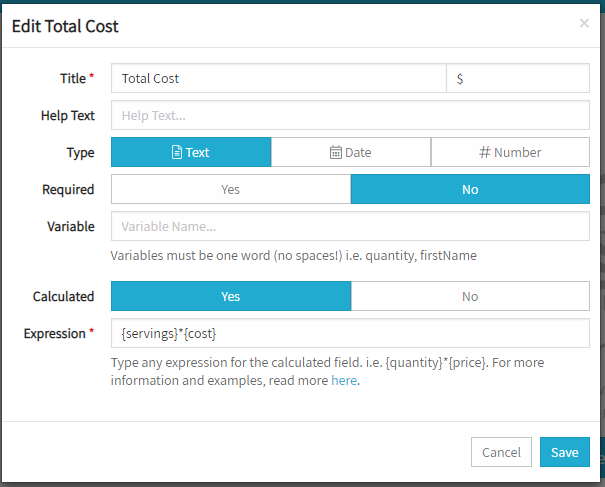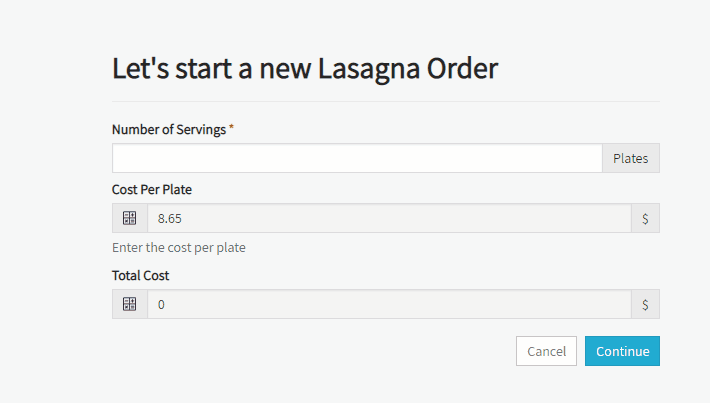# Calculated Fields

In Noviqu's workflow module you can create custom input fields. These fields have the ability to be calculated.

Note: When creating an input field for a workflow you must be on the edit screen and the workflow must be unpublished.

Calculated fields can work on any of the data types available which are:

• Text
• Date
• Number

In order to use a previous input in your calculation you must have given it a variable name. Variable names must be one word, no spaces and wrapped in "{}" brackets. Below are a few examples of how you could use calculated fields.

### Calculating cost based off of quantity.

In this example we are going to calculate total cost based off of per item cost and the quantity of items. We will have two inputs:

• Number of Servings
• Cost Per Plate

These will be used to calculate the total order cost. The equation or expression is essentially Number of Servings X Cost Per Plate. First lets create the Number of Servings input.Next we will add the calculated field cost per plate.Finally we need to put it all together in the Total Cost input.Above what we did was create three inputs. Two are calculated and one is user input.  We can calculate the total cost by taking servings * cost. You must wrap the variables in "{}" brackets for the system to know they are variables. Below is the outcome when a user is working an actual instance of the workflow.### Calculating Dates

Calculating Dates is a little more complicated. Noviqu uses a library called moment.js to work with dates. You can find out more here. Here are a few example equations.